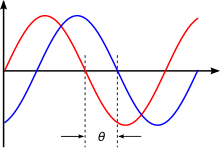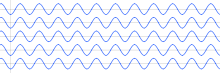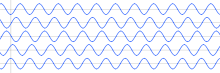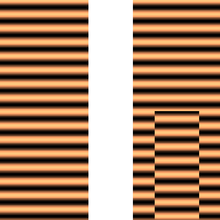# Phase (waves)

﻿
Phase (waves)

Phase in waves is the fraction of a wave cycle which has elapsed relative to an arbitrary point.

## Formula

The phase of an oscillation or wave refers to a sinusoidal function such as the following:$x(t) = A\cdot \cos( 2 \pi f t + \theta )\,$$y(t) = A\cdot \sin( 2 \pi f t + \theta ) = A\cdot \cos( 2 \pi f t + \theta -\pi/2),\,$

where A, f, and$\theta$ are constant parameters. These functions are periodic with period$T = 1/f$, and they are identical except for a displacement of$T/4$ along the$t$ axis. The term phase can refer to several different things:

• It can refer to a specified reference, such as$\cos( 2 \pi f t)\,$, in which case we would say the phase of$x(t)$ is$\theta$, and the phase of$y(t)$ is$\theta -\pi/2$.
• It can refer to$\theta$, in which case we would say$x(t)$ and$y(t)$ have the same phase but are relative to different references.
• In the context of communication waveforms, the time-variant angle$2 \pi f t + \theta,\,$  or its modulo$2\pi$ value, is referred to as instantaneous phase, but often just phase.  Instantaneous phase has a formal definition that is applicable to more general functions and unambiguously defines a function's initial phase at t=0.  Accordingly, it is$\theta$ for$x(t)$  and$\theta -\pi/2$  for$y(t)$.  (also see phasor)

## Phase shiftIllustration of phase shift. The horizontal axis represents an angle (phase) that is increasing with time.

Phase shift is any change that occurs in the phase of one quantity, or in the phase difference between two or more quantities.$\theta$  is sometimes referred to as a phase-shift, because it represents a "shift" from zero phase. But a change in$\theta$ is also referred to as a phase shift.

For infinitely long sinusoids, a change in$\theta$ is the same as a shift in time, such as a time-delay.  If$x(t)$ is delayed (time-shifted) by$\begin{matrix} \frac{1}{4} \end{matrix}$  of its cycle, it becomes:$x(t - \begin{matrix} \frac{1}{4} \end{matrix}T) \,$$= A\cdot \cos(2 \pi f (t - \begin{matrix} \frac{1}{4} \end{matrix}T) + \theta) \,$$= A\cdot \cos(2 \pi f t - \begin{matrix}\frac{\pi }{2} \end{matrix} + \theta ),\,$

whose "phase" is now$\theta - \begin{matrix}\frac{\pi }{2} \end{matrix}.$   It has been shifted by$\begin{matrix}\frac{\pi }{2} \end{matrix}$ radians.

## Phase differenceIn-phase wavesOut-of-phase wavesLeft: the real part of a plane wave moving from top to bottom. Right: the same wave after a central section underwent a phase shift, for example, by passing through a glass of different thickness than the other parts. (The illustration on the right ignores the effect of diffraction, which would make the waveform continuous away from material interfaces and would add increasing distortions with distance.).

Phase difference is the difference, expressed in electrical degrees or time, between two waves having the same frequency and referenced to the same point in time. Two oscillators that have the same frequency and different phases have a phase difference, and the oscillators are said to be out of phase with each other. The amount by which such oscillators are out of step with each other can be expressed in degrees from 0° to 360°, or in radians from 0 to 2π. If the phase difference is 180 degrees (π radians), then the two oscillators are said to be in antiphase. If two interacting waves meet at a point where they are in antiphase, then destructive interference will occur. It is common for waves of electromagnetic (light, RF), acoustic (sound) or other energy to become superposed in their transmission medium. When that happens, the phase difference determines whether they reinforce or weaken each other. Complete cancellation is possible for waves with equal amplitudes.

Time is sometimes used (instead of angle) to express position within the cycle of an oscillation.

• A phase difference is analogous to two athletes running around a race track at the same speed and direction but starting at different positions on the track. They pass a point at different instants in time. But the time difference (phase difference) between them is a constant - same for every pass since they are at the same speed and in the same direction. If they were at different speeds (different frequencies), the phase difference is undefined and would only reflect different starting positions. Technically, phase difference between two entities at various frequencies is undefined and does not exist.
• Time zones are also analogous to phase differences.

## In-phase and quadrature (I&Q) components

The term in-phase is also found in the context of communication signals:$A(t)\cdot \sin[2\pi ft + \phi(t)] = I(t)\cdot \sin(2\pi ft) + Q(t)\cdot \underbrace{\cos(2\pi ft)}_{\sin\left(2\pi ft + \frac{\pi}{2} \right)}$

and:$A(t)\cdot \cos[2\pi ft + \phi(t)] = I(t)\cdot \cos(2\pi ft) \underbrace{{}- Q(t)\cdot \sin(2\pi ft)}_{{} + Q(t)\cdot \cos\left(2\pi ft + \frac{\pi}{2}\right)},$

where$\ f\,$ represents a carrier frequency, and$I(t)\ \stackrel{\text{def}}{=}\ A(t)\cdot \cos\left(\phi(t)\right), \,$$Q(t)\ \stackrel{\text{def}}{=}\ A(t)\cdot \sin\left(\phi(t)\right).\,$$A(t)\,$ and$\phi(t)\,$ represent possible modulation of a pure carrier wave, e.g.:$\sin(2\pi ft)\,$ (or$\cos(2\pi ft).\,$) The modulation alters the original$\sin\,$ (or$\cos\,$) component of the carrier, and creates a (new)$\cos\,$ (or$\sin\,$) component, as shown above. The component that is in phase with the original carrier is referred to as the in-phase component. The other component, which is always 90° ($\begin{matrix} \frac{\pi}{2} \end{matrix}$ radians) "out of phase", is referred to as the quadrature component.

## Phase coherence

Coherence is the quality of a wave to display well defined phase relationship in different regions of its domain of definition.

In physics, quantum mechanics ascribes waves to physical objects. The wave function is complex and since its square modulus is associated with the probability of observing the object, the complex character of the wave function is associated to the phase. Since the complex algebra is responsible for the striking interference effect of quantum mechanics, phase of particles is therefore ultimately related to their quantum behavior.

## Phase compensationExample of a phase compensation circuit.

Phase compensation is the correction of phase error (i.e., the difference between the actually needed phase and the obtained phase). A phase compensation is required to obtain stability in an opamp. A capacitor/RC network is usually used in the phase compensation to keep a phase margin. A phase compensator subtracts out an amount of phase shift from a signal which is equal to the amount of phase shift added by switching one or more additional amplifier stages into the amplification signal path.

Wikimedia Foundation. 2010.

### Look at other dictionaries:

• Phase contrast microscopy — Phase contrast image of a cheek epithelial cell Phase contrast microscopy is an optical microscopy illumination technique of great importance to biologists in which small (invisible to the human eye) phase shifts in the light passing through a… …   Wikipedia

• Phase angle — In the context of vectors and phasors, the term phase angle refers to the angular component of the polar coordinate representation. The notation Aang ! heta, for a vector with magnitude (or amplitude ) A and phase angle θ, is called angle… …   Wikipedia

• Phase — A phase is one part or portion in recurring or serial activities or occurrences logically connected within a greater process, often resulting in an output or a change. Phase or phases may also refer to:In science and engineering* Phase (matter),… …   Wikipedia

• Phase distortion synthesis — is a synthesis method introduced in 1984 by Casio in its CZ range of synths, and similar to Frequency modulation synthesis in the sense that they are both built on phase modulation. Basically a sine wave is played, but by modifying the phase… …   Wikipedia

• phase — phase1 [fāz] n. [ModL phasis < Gr < phainesthai, to appear, akin to phainein: see FANTASY] 1. any of the recurrent stages of variation in the illumination and apparent shape of a moon or a planet 2. any of the stages or forms in any series… …   English World dictionary

• phase-contrast microscope — [fāz′kän′trast΄] n. a compound microscope that uses special lenses, diaphragms, etc. to alter slightly the paths of light waves from an object, thus producing a diffraction pattern that allows the viewing of transparent parts: also phase… …   Universalium

• phase-contrast microscope — [fāz′kän′trast΄] n. a compound microscope that uses special lenses, diaphragms, etc. to alter slightly the paths of light waves from an object, thus producing a diffraction pattern that allows the viewing of transparent parts: also phase… …   English World dictionary

• Phase-shift keying — Passband modulation v · d · e Analog modulation AM · …   Wikipedia

• phase — phaseless, adj. phasic, phaseal, adj. /fayz/, n., v., phased, phasing. n. 1. any of the major appearances or aspects in which a thing of varying modes or conditions manifests itself to the eye or mind. 2. a stage in a process of change or… …   Universalium

• Phase velocity — periodic gravity waves on the surface of deep water. The red dot moves with the phase velocity, and is located at a fixed wave phase: the crest for the case shown.The phase velocity (or phase speed) of a wave is the rate at which the phase of the …   Wikipedia